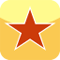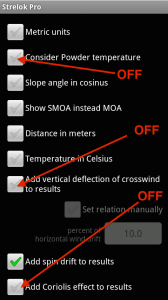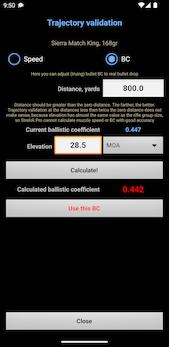﻿ Configuring and tuning Strelok Pro [Android]

#Configuring and tuning Strelok Pro [Android]

1. Enter Scope parameters

2. Enter Cartridge parameters.

3. Measure muzzle speed at different temperatures and input "speed-temperature" pairs to the table. . The difference between the temperatures must be at least 15 degrees. From this table Strelok Pro will calculate your powder sensitivity factor and muzzle speed at current temperature.  Important! Strelok Pro can calculate powder temperature sensitivity factor if you enter at least 2 pairs "speed-temperature"!

You can to tap the button ‘Generate second pair’ if you have one pair ’speed-temperature’ entered. In this case Strelok Pro will guess what values second pair can have according common powder temperature sensitivity.

4. In the begining, turn OFF Consider Powder Temperature, Coriolis effect accounting and Vertical deflection from cross-wind. You can turn it ON later.5. Zero your rifle and save you zeroing weather.

7. Now you are ready to shoot.

Recommended: Make tall test of your scope as described here:

Shoot to 800 yards distance

8. Do 'truing' by ballistic coefficient if needed. Use G7 ballistic coefficient if possible!
Shoot at distance bigger than zero distance. Assume, this is 800 yards (Distance should be greater than the zero distance. The farther, the better). Calculated elevation for this distance is 28.3 MOA
Find out REAL elevation is needed to hit the target. Assume, this is 28.5 MOA
On the trajectory validation screen enter Elevation as 28.5 MOA
Tap button "Calculate" and ballistic coefficient at which elevation will be equal 28.5 MOA will be calculated. It this case, it will be 0.442
Tap button "Use this BC" and this calculated ballistic coefficient will replace your current ballistic coefficient (0.447->0.442) and screen will be closed.On the main screen tap "Calculate" button and you will get calculated elevation which equals REAL elevation - 28.5 MOA
Now you can input any distance and hit the target.